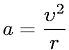Equations > Physics > Mechanics > Centripetal acceleration

### Centripetal accelerationLatex Code:

MathML Code:

 $a=\frac{\upsilon }^{2}r$

MathType 5.0: